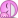# Gauss' Law symmetry of charge distribution

This is a general question, I was reading my textbook and this statement confuses me:

"The symmetry of the electric field must match the symmetry of the charge distribution"
this is said regarding symmetry in relation to GAuss' law.
I do not understand what they mean by symmetry of charge distribution. They say only a cylinder has the same symmetry of charge dist. and electric field, but I fail to understand "what" exactly is the symmetry of charges.

Thanks a lot

## Answers and Replies

collinsmark
Homework Helper
Gold Member
"The symmetry of the electric field must match the symmetry of the charge distribution"
this is said regarding symmetry in relation to GAuss' law.
Thanks a lot

I'm not really sure what that means either. Perhaps if there was more context, I'd have a better answer.

But allow me to speculate about what the textbook might be saying. Gauss' law is only useful in situations involving symmetry. Don't misunderstand, Gauss' law always holds true no matter what the situation, but it just isn't very useful unless there is symmetry. You can use a spherical Gaussian surface for things that have spherical symmetry (including point charges and spheres), a cylindrical Gaussian surface for things with infinitely long cylindrical symmetry (including infinite line charges and cylinders), and a Gaussian "pillbox" surface for things with infinite planar symmetry. Just make sure that the Gaussian surface that you choose matches your charge distribution, in terms of symmetry. In other words, don't use a spherical Gaussian surface to calculate the electric field of an infinite line charge (use a cylindrical surface for that).

Gauss' law is wonderful to use if the charge distribution you are working with has these symmetry properties. The trick is you need to find a Gaussian surface such that the electric field's magnitude is constant on the surface. But if you can't find such a surface, Gauss' law probably won't do you much good, and you will have to use an alternate method.

hey thanks a lot
I understand what you're saying but what I essentially dont understand is generally what is the symmetry of charge? like you mention how we shouldn't choose spherical Gaussian surface for calc. of infinite line of charge. but I dont understand how charge distribution has symmetry? Like we can say this charge distribution has a spherical symmetry, but what does that really mean?
thanks a lot again

collinsmark
Homework Helper
Gold Member
Well, if you have a uniformly charged sphere, then at the center of the sphere, the charge distribution looks identical in all directions. You could rotate the sphere around in any direction and it won't change anything. So that's an example of spherical symmetry.

A uniformly charged, infinitely long cylinder has a symmetry too. Namely you can twirl the cylinder around its axis, and it won't change anything.

Now imagine a sphere that is not uniformly charged. Suppose there is more charge on one side of the sphere than the other. This is a situation where Gauss' law probably won't be very useful in calculating the electric field.

As it happens, Gauss' law isn't very useful when calculating the electric field of a finite length cylinder either -- even if the charge is distributed evenly. This is because it is not so easy to think up a Gaussian surface that would have a constant electric field strength over its surface. So even though a finite length cylinder has certain symmetrical properties, Gauss' law still isn't very useful with it.

About the only things you ever use with Gauss' law are uniformly charged: spheres, spherical shells, points, infinitely long lines, infinitely long cylinders, and infinitely long planes. By that I mean using it to calculate the electric fields. It's still always valid in any charge distribution. And if you know the electric field, you can always use it to find the enclosed charge. But when starting with a known charge distribution and deriving the electric field, it usually only useful in these situations.

Thanks a lotD